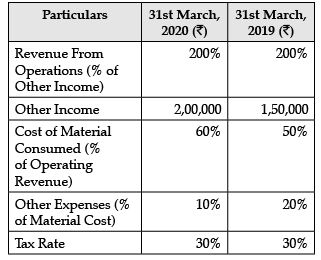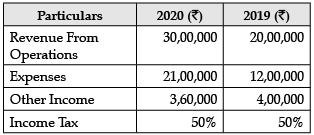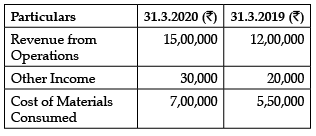# Test: Analysis of Financial Statements- Case Based Type Questions

## 12 Questions MCQ Test Accountancy Class 12 | Test: Analysis of Financial Statements- Case Based Type Questions

Description
Attempt Test: Analysis of Financial Statements- Case Based Type Questions | 12 questions in 24 minutes | Mock test for Commerce preparation | Free important questions MCQ to study Accountancy Class 12 for Commerce Exam | Download free PDF with solutions
QUESTION: 1

### Read the following information and answer the given questions:The following data is available from Pitambar Ltd.Q. What is the Net Profit after Tax in March 2020?

Solution: Net Profit before Tax = ₹4,00,000 + ₹2,00,000 - ₹2,40,000 - ₹24,000 = ₹3,36,000

Tax = ₹3,36,000 x 30 / 100 = ₹1,00,800

Net Profit after Tax = ₹3,36,000 - ₹1,00,800 = ₹2,35,200

QUESTION: 2

### Read the following information and answer the given questions:The following data is available from Pitambar Ltd.Q. What is the percentage of the Total Revenue from Revenue from operations in 2019?

Solution: Revenue from operations = 200% of ₹1,50,000 = ₹3 ,00,000

Total Revenue = ₹3,00,000 + ₹1,50,000 = ₹4 ,50,000

Required Percentage = 4,50,000 / 3,00,000 × 100 = 150%

QUESTION: 3

### Read the following information and answer the given questions:The following data is available from Pitambar Ltd.Q. What is the revenue from operations on 31st March, 2020?

Solution: 200% of ₹2,00,000 = ₹4,00,000
QUESTION: 4

The following data is available from Pitambar Ltd.Q. What is the value of the tax paid by the firm in 2019?

Solution: Tax Paid = (₹3,00,000 + ₹1,50,000 - ₹1,50,000 - ₹30,000) × 30 / 100

= ₹2,70,000 × 30 / 100

= ₹81,000

QUESTION: 5

Following information are taken from the books of Agarwal Pvt Ltd.:Q. What is the percentage change in the tax paid?

Solution: Net Profit before Tax for 2020 = ₹12,60,000

2019 = ₹12,00,000

Tax paid for 2020 = ₹6,30,000

2019 = ₹6 ,00,000

Percentage Change = ₹30,000 / ₹6 ,00,000 x 100 = 5%

QUESTION: 6

Following information are taken from the books of Agarwal Pvt Ltd.:Q. What is the percentage change in the profit earned after tax?

Solution: Net Profit after Tax for 2020 = ₹6,30,000

2019 = ₹6,00,000

Percentage Change = ₹30,000 / ₹6 ,00,000 x 100 = 5%

QUESTION: 7

Following information are taken from the books of Agarwal Pvt Ltd.:Q. What is the value of absolute change in the Total Revenue?

Solution: Total Revenue = Revenue from operations + Other Income

For 2019 = ₹24,00,000

For 2020 = ₹33,60,000

Absolute Change = ₹24,00,000 - ₹33,60,000 = ₹9,60,000

QUESTION: 8

Following information are taken from the books of Agarwal Pvt Ltd.:Q. The absolute change in the Expenses is:

Solution: ₹21,00,000 - ₹12,00,000 = ₹9,00,000
QUESTION: 9

Following is the data given of Lalit Ltd.Q. What is the percentage change in the Total Expenses?

Solution: 1,50,000 / 5,50,000 = 27.27%
QUESTION: 10

Following is the data given of Lalit Ltd.Q. What is the percentage change in the Total Revenue earned?

Solution: 3,00,000 + 10,000 / 12,00,000+20,000 x 100 = 24.81%
QUESTION: 11

Following is the data given of Lalit Ltd.Q. What is the percentage change in the Revenue from operations?

Solution: 3,00,000 / 12,00,000 x 100 = 25%
QUESTION: 12

Following is the data given of Lalit Ltd.Q. What is the change in the profit before tax?

Solution: Total Revenue in 2013 = ₹12,00,000 + ₹20,000 = ₹12,20,000

Total Expenses = ₹5 ,50,000

Profit = ₹1 2,20,000 - ₹5,50,000 = ₹6,70,000

In 2014, Total Revenue = ₹15,00,000 + ₹30,000 = ₹15,30,000

Total Expenses = ₹7,00,000

Profit = ₹1 5,30,000 - ₹7,00,000 = ₹8,30,000

Change in profit = ₹8,30,000 - ₹6,70,000 = ₹1,60,000Use Code STAYHOME200 and get INR 200 additional OFF Use Coupon Code